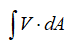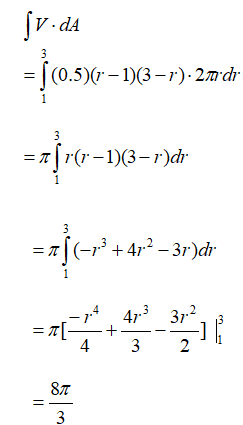# A solid rod of radius 1 cm is placed in a pipe of radius 3 cm so that their axes are aligned. Water flows through the pipe and around the rod. Find the flow rate if the velocity of the water is given by the radial function v(r) = 0.5(r − 1)(3 − r) cm/s.

Question
1 views

A solid rod of radius 1 cm is placed in a pipe of radius 3 cm so that their axes are aligned. Water flows
through the pipe and around the rod. Find the flow rate if the velocity of the water is given by the radial
function v(r) = 0.5(r − 1)(3 − r) cm/s.

check_circle

Step 1

velocity of water  v(r) = 0.5(r − 1)(3 − r) cm/s.

flow rate is given as,Step 2

Limits for r would be from 1 to 3...

### Want to see the full answer?

See Solution

#### Want to see this answer and more?

Solutions are written by subject experts who are available 24/7. Questions are typically answered within 1 hour.*

See Solution
*Response times may vary by subject and question.
Tagged in